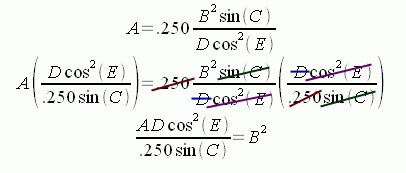SEARCH HOMEMath Central Quandaries & QueriesQuestion from MARK: It has been a long time since I have had to reverse a formula.                     (B^2*SIN(C)  A=.250 *     --------------                      D*COS^2(E) ^=POWER B^2= B SQUARED I need to solve for B. I would really appreciate it.Hi Mark.

If you want to isolate B, then you need to get rid of everything else around it. The way you do that in an expression like yours with multiplications and divisions is by cancelling things out. Whatever you do to one side you must do to the other:Can you solve it from here? You just need to take the square root of both sides and simplify.

Stephen La Rocque.>

PS: Technically, you should make the proviso that sin(C) cannot equal zero. Can you see that if sin(C) equals 0, then what I've done is to divide by zero? That's something that is disallowed, so to prevent it, we just state that sin(C) is not equal to zero. Of course, if you know that A is non-zero, then sin(C) can't be zero anyway!Math Central is supported by the University of Regina and The Pacific Institute for the Mathematical Sciences.